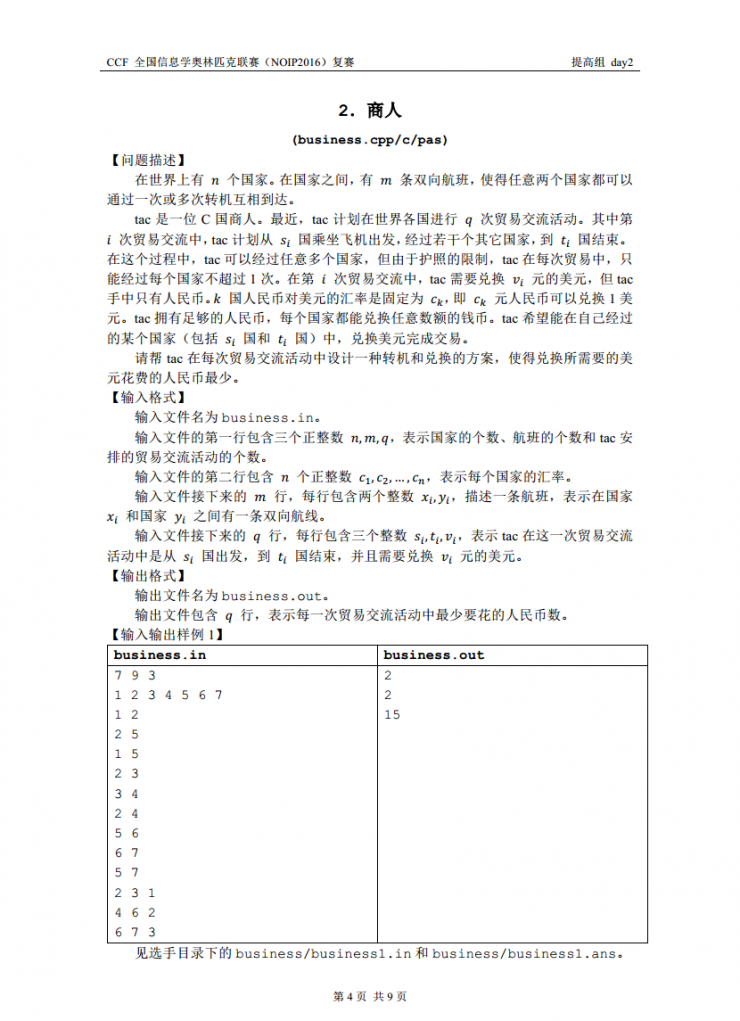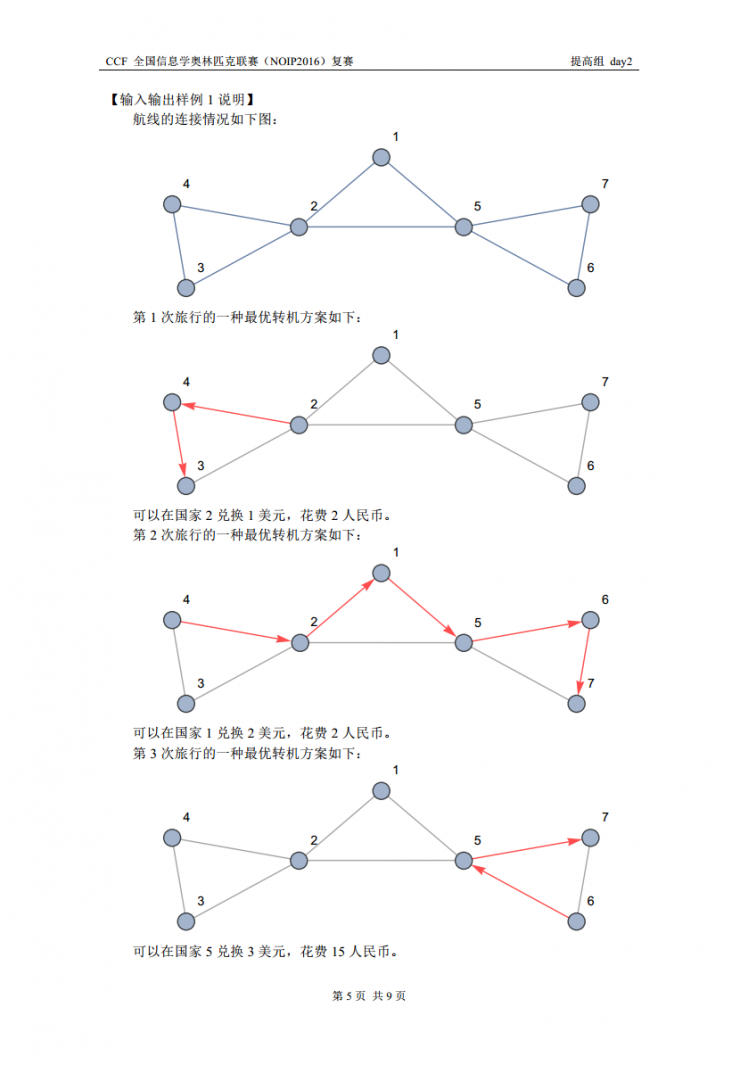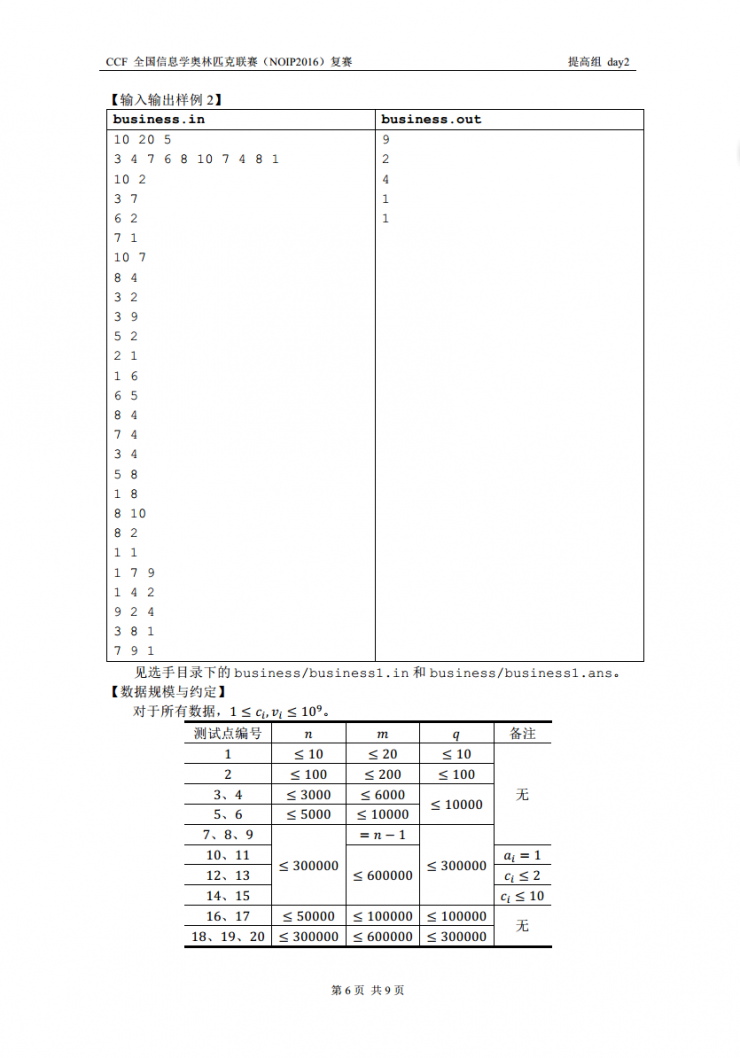# [BJOI2018] 链上二次求和

• ### 题目描述

YJC 有一棵 $$n$$ 个节点的树， $$i$$、$$i+1$$ （$$1 \leq i < n$$）节点间有一条边，第 $$i$$ 个点的权值为整数 $$a_i$$ 。

# YZOJ P4637 [CSP-S 2019 五校联训 Round 2]由比滨结衣(sqrt)

• ### 题目描述

1 l r x 将区间 $$[l,r]$$ 中的所有数变为 $$x$$。

2 l r x 查询区间 $$[l,r]$$ 中数字 $$x$$ 的出现次数。

• ### 样例输出

Source： BZOJ 5104…

…

# YZOJ P3098 [JLOI2016]成绩比较

• ### 题目描述

G系共有 $$n$$ 位同学，$$m$$ 门必修课。这 $$n$$ 位同学的编号为 $$0$$ 到 $$n-1$$ 的整数，其中B神的编号为 $$0$$ 号。

# YZOJ P2791 商人# YZOJ P3195 [NOI2017]游戏

• ### 题目描述

$$n$$ 场游戏的地图可以用一个小写字母组成的字符串描述。例如：$$S=\underline{\mathrm{xaabxcbc}}$$ 表示小 L 计划进行 $$8$$ 场游戏，其中第 $$1$$ 场和第 $$5$$ 场的地图类型是 $$x$$，适合所有赛车，第 $$2$$ 场和第 $$3$$ 场的地图是 $$a$$，不适合赛车 $$A$$，第 $$4$$ 场和第 $$7$$ 场的地图是 $$b$$，不适合赛车 $$B$$，第 $$6$$ 场和第 $$8$$ 场的地图是 $$c$$，不适合赛车 $$C$$ 。

• ### 输入格式

$$n,d,S$$ 的含义见题目描述，其中 $$S$$ 包含 $$n$$ 个字符，且其中恰好 $$d$$ 个为小写字母 $$x$$ 。

…

# YZOJ P3939 [HAOI2016]找相同字符

• ### 样例输出

Source: BZOJ 4566…